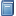Buchempfehlung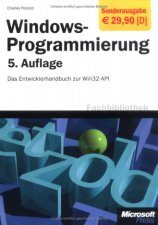Windows-Programmierung. Das Entwicklerhandbuch zur WIN32-API
"Der" Petzold, das über 1000 Seiten starke Standardwerk zum Win32-API - besonders nützlich u. a. bei der GUI-Programmierung in FreeBASIC! [Mehr Infos...]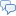FreeBASIC-Chat
Es sind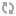Benutzer im FreeBASIC-Chat online.
(Stand:  )

# fb:porticula NoPaste

## GFX2SDL.bi

 Uploader: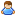OneCypher Datum/Zeit: 02.05.2010 00:26:39
Hinweis: Dieser Quelltext ist Bestandteil des Projekts GFX 2 SDL, zu dem es auf FreeBASIC-Portal.de eine Projektseite gibt.

```#include "SDL\SDL.bi" namespace SDL     #include "crt.bi"     Dim Shared SDLMutex as any ptr     Dim Shared SDLKeyCond as any ptr     Dim Shared SDLMouseCond as any ptr     Sub GetSDLMouse(byref mx as integer=0,byref my as integer=0,byref ms as integer=0,byref mb as integer=0)             dim tmpx1 as integer             dim tmpy1 as integer             dim SDLMButton as ubyte             SDLMButton = SDL_GetMouseState(@mx, @my): mb = 0             if (SDLMButton and SDL_BUTTON(SDL_BUTTON_LEFT)) then mb = mb + 1             if (SDLMButton and SDL_BUTTON(SDL_BUTTON_RIGHT)) then mb = mb + 2             'Folgendes klappt leider bisher nicht :-(((             'if (SDLMButton AND SDL_BUTTON(SDL_BUTTON_WHEELUP)) then ms = 1             'if (SDLMButton AND SDL_BUTTON(SDL_BUTTON_WHEELDOWN)) then ms = -1             SDL_PumpEvents     end sub     function SDLInkey(SEvent as any ptr) as string         DIM SDLEvent as SDL_Event ptr = SEvent         Dim Tmp as string         if SDL_PollEvent ( SDLEvent ) then             if SDLEvent->type = SDL_KEYDOWN then                 with *SDLEvent                     if .Key.KeySym.sym > 126 then                         return CHR(255, .key.keysym.scancode)                     else                         TMP = CHR(.key.keysym.unicode_ )                     end if                     'if .key.keysym.mod_ = 4097 then Tmp = ucase(Tmp)                     if ( .key.keysym.mod_ = 4160 or .key.keysym.mod_ = 4224) and CHR(.key.keysym.sym) = "v" then tmp = CHR(22)                     if ( .key.keysym.mod_ = 4160 or .key.keysym.mod_ = 4224) and CHR(.key.keysym.sym) = "c" then tmp = CHR(3)                 end with                 return TMP             else                 return ""             end if         end if     end function     sub Buffer2Surface(MyBuffer as any ptr, Srfc as SDL_Surface ptr)         dim MyPixData as any ptr         Dim ImgSize as uinteger         DIm as integer iw, ih         dim as uinteger p         imageinfo MyBuffer,iw,ih,,p,MyPixdata, ImgSize         SDL_LockSurface( Srfc )         if Srfc->pitch > p then             if Srfc->h > ih then                 for y as integer = 0 to ih -1                     memcpy Srfc->pixels + (y * Srfc->pitch), MyPixData + (y*p), p                 next             else                 for y as integer = 0 to Srfc->h -1                     memcpy Srfc->pixels + (y * Srfc->pitch), MyPixData + (y*p), p                 next             end if         end if         if Srfc->pitch < p then             if Srfc->h > ih then                 for y as integer = 0 to ih -1                     memcpy Srfc->pixels + (y * Srfc->pitch),MyPixData + (y*p) , Srfc->pitch                 next             else                 for y as integer = 0 to Srfc->h -1                     memcpy Srfc->pixels + (y * Srfc->pitch),MyPixData + (y*p) , Srfc->pitch                 next             end if         end if         if Srfc->pitch = p then             if Srfc->h > ih then                 for y as integer = 0 to ih -1                     memcpy Srfc->pixels + (y * Srfc->pitch),MyPixData + (y*p) , Srfc->pitch                 next             else                 memcpy Srfc->pixels, MyPixData, Srfc->pitch * Srfc->h 'ImgSize - 32             end if         end if         SDL_Flip Srfc         SDL_UnlockSurface( Srfc )     end sub     Dim Shared SrfcPtr as SDL_Surface ptr     Dim Shared ClrDepth as integer     Dim Shared BufferPtr as any ptr     Dim Shared ThreadPtr as any ptr     Dim shared FlagModes as uinteger     Dim Shared SDLEvent as SDL_Event     Dim Shared FrameRate as integer     Dim shared in as string     Dim Shared MouseX as integer     Dim Shared MouseY as integer     Dim Shared MouseScroll as integer     Dim Shared MouseButton as integer     Dim Shared Resized as integer     Dim Shared NewWidth as integer     Dim Shared NewHeight as integer     Dim shared BufferPtrPtr as any ptr ptr     Sub SDLThread()         '#define SDLDebug         Dim T1 as double         #ifdef SDLDebug             Dim t2 as double             Dim FrameCounter as double             Dim LastFPS as double         #endif         DIm as integer iw1, ih1         DIm as integer iw2, ih2         imageinfo *BufferPtrPtr,iw1,ih1         mutexlock SDLMutex             if SrfcPtr = 0 then                 SrfcPtr = SDL_SetVideoMode(iw1, ih1, ClrDepth, SDL_SWSURFACE + FlagModes)                 SDL_EnableKeyRepeat(200, 10)                 SDL_EnableUNICODE( SDL_ENABLE )             end if         Mutexunlock SDLMutex         dim t as string         t1 = timer         #ifdef SDLDebug             t2 = timer         #endif         While SrfcPtr <> 0             mutexlock SDLMutex                 if SrfcPtr = 0 then                     ThreadPtr = 0                     exit sub                 end if                 #ifdef SDLDebug                 if timer > t2 +1 then                     LastFPS = FrameCounter                     FrameCounter = 0                     t2 = timer                 end if                 #endif                 imageinfo *BufferPtrPtr,iw2,ih2                 if iw2 <> iw1 or ih2 <> ih1 then                     SrfcPtr = SDL_SetVideoMode(iw2, ih2, ClrDepth, SDL_SWSURFACE + FlagModes)                     iw1 = iw2: ih1 = ih2                 end if                 if SDLEvent.type = SDL_VIDEORESIZE then                     if SDLEvent.resize.w <> iw1 or SDLEvent.resize.h <> ih1 then                         NewWidth = SDLEvent.resize.w                         NewHeight = SDLEvent.resize.h                         Resized = (1 <> 0)                     end if                 end if                 if timer >= t1 + (1 / FrameRate) then                     #ifdef SDLDebug                     FrameCounter = FrameCounter +1                     draw string *BufferPtrPtr, (-1,0), "Frames p/s=" & int(LastFPS),RGB(0,0,0)                     draw string *BufferPtrPtr, (+1,0), "Frames p/s=" & int(LastFPS),RGB(0,0,0)                     draw string *BufferPtrPtr, (0,-1), "Frames p/s=" & int(LastFPS),RGB(0,0,0)                     draw string *BufferPtrPtr, (0,+1), "Frames p/s=" & int(LastFPS),RGB(0,0,0)                     draw string *BufferPtrPtr, (0,0), "Frames p/s=" & int(LastFPS),RGB(255,255,255)                     #endif                     Buffer2Surface *BufferPtrPtr, SrfcPtr                     t1 = timer                 end if                 if SDLEvent.type = SDL_QUIT_ then                     in = CHR(255) & CHR(107)                 else                     in = SDLInkey(@SDLEvent)                 end if                 CondBroadCast SDLKeyCond                 GetSDLMouse( MouseX,MouseY,MouseScroll,Mousebutton)                 CondBroadCast SDLMouseCond             Mutexunlock SDLMutex         Wend     End sub     Sub FitBuffer()         Dim OldBuffer as any ptr = *BufferPtrPtr         *BufferPtrPtr = ImageCreate(NewWidth, NewHeight, RGB(0,0,0))         ImageDestroy OldBuffer         Resized = (1 <> 1)     end sub     Sub ScreenRes_tmp(Screen_Width as integer, Screen_Height as integer, ClrDepth1 as integer = 32, Pages as integer = 1, Flags as uinteger = 0, FrameRate1 as integer= 30)         DIM driver AS STRING         Dim OldBuffer as any ptr         if BufferPtrPtr = 0 then BufferPtrPtr = @BufferPtr         if SDLMutex = 0 then SDLMutex = MutexCreate         If SDLKeyCond = 0 then SDLKeyCond = CondCreate         if SDLMouseCond = 0 then SDLMouseCond = CondCreate         'Mutexlock SDLMutex         FlagModes = 0         FrameRate = FrameRate1         ClrDepth = ClrDepth1         SCREENINFO , , , , , , driver         if driver = "" then ScreenRes Screen_Width, Screen_Height, ClrDepth, , -1         if Flags AND &H01 then FlagModes = FlagModes + SDL_FULLSCREEN         if Flags AND &H02 then FlagModes = FlagModes         if Flags AND &H04 then FlagModes = FlagModes         if Flags AND &H08 then FlagModes = FlagModes + SDL_NOFRAME         if Flags AND &H10 then FlagModes = FlagModes         if Flags AND &H20 then FlagModes = FlagModes         if Flags AND &H40 then FlagModes = FlagModes         if Flags AND &H80 then FlagModes = FlagModes         if Flags AND &H100 then FlagModes = FlagModes + SDL_RESIZABLE         OldBuffer = *BufferPtrPtr         *BufferPtrPtr = ImageCreate(Screen_Width, Screen_Height, RGB(0,0,0))         if OldBuffer <> 0 then ImageDestroy OldBuffer         If ThreadPtr = 0 then ThreadPtr = ThreadCreate(cast(any ptr, @SDLThread))     end sub     Sub Screen(Modus as integer, ClrDepth1 as integer = 32, Pages as integer = 1, Flags as uinteger = 0, FrameRate1 as integer= 30)         Dim w as integer, h as integer         Select Case Modus         case -1             ScreenControl 3, w, h             FlagModes = FlagModes + SDL_FULLSCREEN         case 0             SDL_Quit             SrfcPtr = 0             if *BufferPtrPtr <> 0 then ImageDestroy *BufferPtrPtr             *BufferPtrPtr = 0             exit sub         Case 14             w = 320: h = 240         Case 15             w = 400: h = 300         Case 16             w = 512: h = 384         Case 17             w = 640: h = 400         Case 18             w = 640: h = 480         Case 19             w = 800: h = 600         case 20             w = 1024: h = 768         case 21             w = 1280: h = 1024         case 22             w = 1600: h = 1200         End select         ScreenRes_tmp(w, h , ClrDepth1 , Pages , Flags , FrameRate1)     End Sub     Sub ScreenRes(Screen_Width as integer, Screen_Height as integer, ClrDepth1 as integer = 32, Pages as integer = 1, Flags as uinteger = 0, FrameRate1 as integer= 30)         ScreenRes_tmp(Screen_Width, Screen_Height , ClrDepth1 , Pages , Flags , FrameRate1)     end sub     Function Inkey() as string         if ThreadPtr <> 0 then             CondWait SDLKeyCond, SDLMutex             return in         end if     end function     sub GetMouse(byref mx as integer=0,byref my as integer=0,byref ms as integer=0,byref mb as integer=0)         if ThreadPtr <> 0 then             CondWait SDLKeyCond, SDLMutex             mx = MouseX             my = MouseY             ms = MouseScroll             mb = MouseButton         end if     end Sub     sub SetBufferPtr(byref BufferVar as any ptr)         if BufferPtrPtr <> 0 then BufferVar = *BufferPtrPtr         BufferPtrPtr = @BufferVar     end sub End NameSpace```